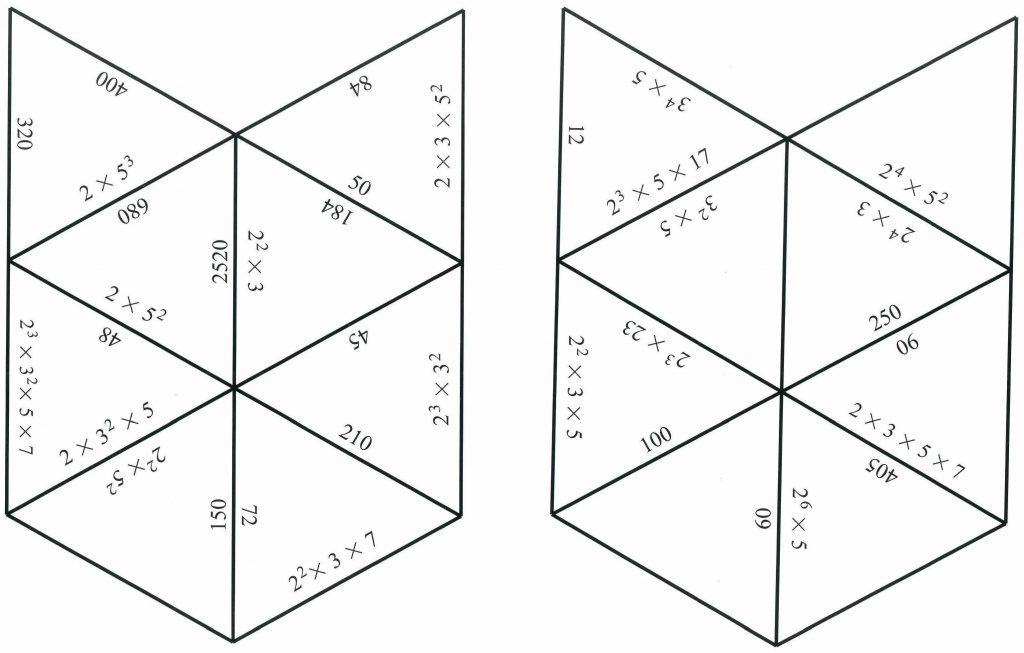Download copies of these shapes here and cut out the 16 small triangles.

Arrange the 16 pieces into a larger triangle so that the numbers on matching edges are the same, for example $$2^2 \times 3 \times 7 = 84$$.

1.Machabaphala John says: